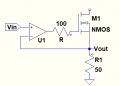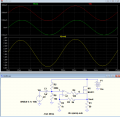# How to drive source follower (with feedback) with an op amp

#### BufkinMK

Joined Apr 28, 2022
7

#### crutschow

Joined Mar 14, 2008
29,768
That circuit looks a little odd.
What is its purpose?
It looks somewhat like a current-drive (constant-current) circuit, but the current will depend upon the MOSFET Vgs threshold voltage, and I would assume you don't want that.

You can't readily substitute an op amp for V2, since that is a floating source.

And that's not a source-follower circuit.
A source-follower output is the MOSFET source.

•BufkinMK

#### BufkinMK

Joined Apr 28, 2022
7
That circuit looks a little odd.
What is its purpose?
It looks somewhat like a current-drive (constant-current) circuit, but the current will depend upon the MOSFET Vgs threshold voltage, and I would assume you don't want that.

You can't readily substitute an op amp for V2, since that is a floating source.

And that's not a source-follower circuit.
A source-follower output is the MOSFET source.
The purpose is to act as a power gain stage for an op amp. It should be in the op amps' feedback loop, which is proving difficult, as you say.

But it effectively functions as a source follower?

#### MrChips

Joined Oct 2, 2009
26,077
•BufkinMK

#### crutschow

Joined Mar 14, 2008
29,768
It should be in the op amps' feedback loop, which is proving difficult, as you say.
Not really.
I just wasn't sure what you were really trying to do.

Below is the basic circuit for an op amp driving a MOSFET as a source-follower.
Some additional compensation parts may be needed for stability, depending on the frequency response you need and the op amp used.•ronsimpson and BufkinMK

#### BufkinMK

Joined Apr 28, 2022
7
Not really.
I just wasn't sure what you were really trying to do.

Below is the basic circuit for an op amp driving a MOSFET as a source-follower.
Some additional compensation parts may be needed for stability, depending on the frequency response you need and the op amp used.

View attachment 266073
Thanks very much. Is it possible to add voltage gain to the op amp stage?

#### BufkinMK

Joined Apr 28, 2022
7
This looks really helpful, thanks very much.

#### BufkinMK

Joined Apr 28, 2022
7
Not really.
I just wasn't sure what you were really trying to do.

Below is the basic circuit for an op amp driving a MOSFET as a source-follower.
Some additional compensation parts may be needed for stability, depending on the frequency response you need and the op amp used.

View attachment 266073
Can you explain how this circuit produces the bias voltage required to turn on the MOSFET, even at a very small input voltage (much less than the threshold Vgs)?

Thank you

#### BufkinMK

Joined Apr 28, 2022
7
Sure.
The gain below is 1 + R2/R3.

View attachment 266104
This circuit only seems to work for feedback resistors of equal value. Am I missing something?

Thanks very much for the help

#### crutschow

Joined Mar 14, 2008
29,768
Can you explain how this circuit produces the bias voltage required to turn on the MOSFET
The high open-loop gain the of the op amp means the output will go to whatever voltage is needed to turn on the MOSFET, so that the difference between the plus and minus op amp inputs is very near zero volts.
This circuit only seems to work for feedback resistors of equal value.
Why do you say that? What's so special about them being equal value?
It will work for any arbitrary values within the limits of the circuit components and voltage supply.

•BufkinMK

#### crutschow

Joined Mar 14, 2008
29,768
Last edited:
•BufkinMK

#### BufkinMK

Joined Apr 28, 2022
7
The high open-loop gain the of the op amp means the output will go to whatever voltage is needed to turn on the MOSFET, so that the difference between the plus and minus op amp inputs is very near zero volts.
Why do you say that? What's so special about them being equal value?
It will work for any arbitrary values within the limits of the circuit components and voltage supply.
Thank you, I don't know why this wasn't working for me.

Is the 0.2v DC bias on the voltage source is the only reason the MOSFET is in the active (linear) mode of operation? Is there any way to bias the MOSFET without manually selecting the 0.2v DC bias? I have tried a potential divider at the MOSFET gate but that doesn't seem to work.

Thanks so much for your help.

#### crutschow

Joined Mar 14, 2008
29,768
Is the 0.2v DC bias on the voltage source is the only reason the MOSFET is in the active (linear) mode of operation?
Yes.
Otherwise the output would be 0V when the input sinewave is 0V and the bottom half of the sinewave would be clipped.
Is the 0.2v DC bias on the voltage source is the only reason the MOSFET is in the active (linear) mode of operation? Is there any way to bias the MOSFET without manually selecting the 0.2v DC bias? I have tried a potential divider at the MOSFET gate but that doesn't seem to work.
You can't offset the MOSFET gate because you are fighting what the op amp is trying to do (maintain the output equal to the input).
The offset must be done at the op amp input.

You need to read some tutorials on how op amp feedback circuits work, as you seem to not understand that.

If you don't want to bias the source, then you can use a voltage divider to generate the input bias and then use capacitive coupling to block the DC.
That will only work with an AC signal, of course.
Simulation below:

If you only have positive signals, then you don't need to bias the input.

Also note that a single-supply type op amp would be needed in the actual circuit.Last edited: